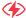# Overview of Some Common Machine Learning Algorithms

Jul 26,2021It is important to have an understanding of machine learning algorithms to be able to take advantage of the most updated and advanced computing technologies. Some of these important algorithms are discussed below:

• Supervised Learning: This algorithm will comprise of a target that must be predicted using given sets of predictors. These given predictors are independent variables and the targets are dependent variables. You can use the independent variables to generate a function which will map these inputs to give outputs. Some examples of supervised learning are Regressions, Random Forest, KNN, Decision Tree and Logistic Regression.

• Unsupervised Learning: This algorithm will not have a target variable which you will need to predict. This algorithm is primarily used for grouping population and therefore used for categorizing customers in various groups. For examples. K-means and Apriori are types of unsupervised learning.

• Reinforcement Learning: By using this third type of algorithm, the machine can make specific decisions. The machine then gets exposed to environments wherein it will self-train using a trial and error method. So, the machine can learn from past mistakes and uses the knowledge thus gained to make better and more accurate business decisions. For example, the Markov Decision Process.

## Common Machine Learning Algorithms

1. Linear Regression:

This algorithm is used for calculating real values such as total sales or cost of houses which are based on continuous variables. You will need the best fit line here to create a relationship between the independent and the dependent variables. This best fit line has been termed as the regression line.

2. Logistic Regression:

This algorithm is meant to calculate discrete values based upon given sets of independent variables. So, it will basically try to predict the probability of an event by fitting specific data to a logical function. This is why it is called logical regression algorithm.

Related Topic:  Do not underestimate the importance of network uptime!

3. Decision Tree:

This is supervised learning algorithm used in classification problems. In this algorithm, the population gets divided into two or even more homogeneous sets. This spilled is carried out based upon important attributes to create distinct groups.

4. SVM (Support Vector Machine):

This algorithm also deals with classification and here every data item is plotted as a point in an n-dimensional space. Here, “n” will stand for the number of features. Value of every feature will be the value of a specific coordinate. The coordinates are called the Support Vectors.

5. Naive Bayes:

This refers to a method for classification which is founded upon the Bayes Theorem. The classifier in Naïve Bayes assumes there is a feature in a class which is not related to the existence of any other feature. This model is easy to create and it is handy for very large datasets.

6. KNN (K- Nearest Neighbors):

This algorithm is used for both regression and classification problems but mainly employed for classification problems. It is simple to use; it stores available cases and classifies the new ones by majority votes.

7. Random Forest:

This refers to an assembly of decision tree where every tree will give a classification. So, the tree “votes” for a specific class and the forest will select the classification which has the maximum number of votes.

8. Dimensionality Reduction Algorithms:

Data capturing has gone up dramatically in the recent years at every stage possible. So, research institutions and governmental agencies are developing new sources for capturing more detailed data. For instance, the e-commerce firms are trying to get additional details about their buyers from their web browsing history, what products they prefer or their purchase history.

Of these, the first type is GBM which is a type of boosting algorithm. This is used when you are handling a lot of data and you have to make predictions with very high prediction power. Boosting will involve a collection of learning algorithms. These combine predictions of various base estimators to increase robustness over one estimator. The second type of boosting algorithm is the XGBoost which has very high predictive power and can make the best choices for accuracy during events. It has both the tree learning algorithm and linear model algorithm which makes this much quicker than other gradient boosting techniques. The third type is the LightGBM which uses tree-based learning algorithm and can guarantee the better accuracy, faster speed, and efficiency, handling of large-scale data etc. Finally, there is the CatBoost algorithm which is very recent and open-source, machine learning algorithm. This is capable of integrating with other deep-learning frameworks such as Apple’s Core ML. it will not need a lot of training as compared to other machine learning models and it can run on different types of data formats.

Subscribe
Notify ofInline Feedbacks

## AWS Standard Consulting Partner

••## Alibaba Cloud## Cyfuture Ltd.

The Cricket Barn
Tiverton
Exeter
EX16 8ND

Ph:   1-888-795-2770
E-mail:   [email protected]